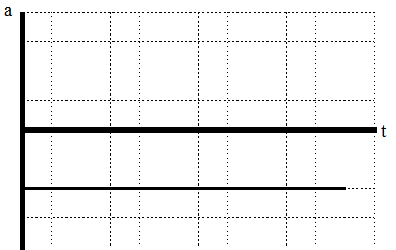$$\require{cancel}$$

# 03. Drawing Motion Graphs in 1D

•• Contributed by Paul D'Alessandris
• Professor (Engineering Science and Physics) at Monroe Community College

# Drawing Motion Graphs

Another useful way to describe the motion of an object is by constructing graphs of the object’s position, velocity, and acceleration vs. time. A graphical representation is a very effective means of presenting information concerning an object’s motion, and, moreover, it is relatively easy to construct motion graphs if you have a correct motion diagram.

Examine the same situation as before:

Template:ExampleStart

The driver of an automobile traveling at 15 m/s, noticing a red-light 30 m ahead, applies the brakes of her car until she stops just short of the intersection.The verbal representation of the situation has already been translated into a motion diagram. A careful reading of the motion diagram allows the construction of the motion graphs.

Template:ExampleEnd

# Drawing the position vs. time graph

We already know, from the motion diagram, that the car starts at position zero, then has positive, increasing positions throughout the remainder of its motion. This information can be transferred onto a position vs. time graph.Notice that the position is zero when the time is equal to zero, the position is always positive, and the position increases as time increases. Also note that in each subsequent second, the car changes its position by a smaller amount. This leads to the graph of position vs. time gradually decreasing in slope until it achieves a slope of zero. Once the car stops, the position of the car should not change.

# Drawing the velocity vs. time graph

From the motion diagram, we know that the velocity of the car is always positive, starts large in magnitude, and decreases until it is zero. This information can be transferred onto a velocity vs. time graph.How do we know that the slope of the line is constant? The slope of the line represents the rate at which the velocity is changing, and the rate at which the velocity is changing is termed the acceleration. Since in this model of mechanics we will only consider particles undergoing constant acceleration, the slope of a line on a velocity vs. time graph must be constant.

# Drawing the acceleration vs. time graph

From the motion diagram, the acceleration of the car can be determined to be negative at every point. Again, in this pass through mechanics, we will only be investigating scenarios in which the acceleration is constant. Thus, a correct acceleration vs. time graph is shown below.